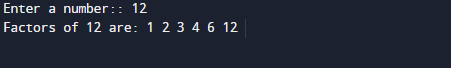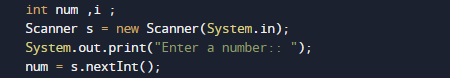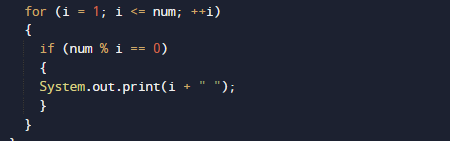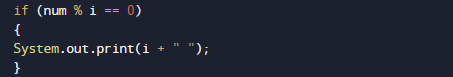# Java Program to Display Factors of a Number

In this tutorial you will learn about the Java Program to Display Factors of a Number and its application with practical example.

In this tutorial, we will learn to create a Java program that will Display Factors of a Number   using Java programming.

## Prerequisites

Before starting with this tutorial we assume that you are best aware of the following Java programming topics:

• Java Operators.
• Basic Input and Output
• Class and Object.
• Basic Java programming.
• Java Loops.
• If-else Statements.

## What Is Factor of  a Number?

Factors:=>Factors of a number are that numbers that divide the number completely and remainder of divisor is equal to zero.
Example:- Let say the numbers= 1, 2, 3, 4, 6 and 12  divides the number 12 completely so they are called the factor of 12.

## Java Program to Display Factors of a Number

In our program we will Find factors of a given Number.Firstly we declare required header file and variable and also initiates required values in variable. Next we take value from user and find all possible factors of a number.

## OutputIn our program  we will take a positive integer value from user by prompting user and with the help of loop and if else statement  we will displays all the positive factors of that number.In the above program, we have first declared and initialized a set variables required in the program.

• num = It will hols number given by user.
• i= for iteration of loop and finding  factor.Logic behind finding factors of a given number is we that we run a for loop from 1 to number and within the loop increment the value of  by 1 in each iteration.
The loop structure of for loop should look like this.Within the for loop  in each iteration we will check the condition for i is a factor of number or not. To find factor we use divisibility of number by using  modulo operator(%).i.e. if( num % i == 0) then i is a factor of number .
If i is a factor of number then we will print the value of i.So we will get the required factors of given number repeatably repeating this process thought out the loop to get all the factors .

In this tutorial we have learn about the Java Program to Display Factors of a Number and its application with practical example. I hope you will like this tutorial.